# 15.1 Standing waves in wind instruments

 Page 1 / 1

## Standing waves in wind instruments

A wind instrument is an instrument that is usually made with a pipe or thin tube. Examples of wind instruments are recorders, clarinets, flutes, organs etc.

When one plays a wind instrument, the air that is pushed through the pipe vibrates and standing waves are formed. Just like with strings, the wavelengths of the standing waveswill depend on the length of the pipe and whether it is open or closed at each end. Let's consider each of the following situations:

• A pipe with both ends open, like a flute or organ pipe.
• A pipe with one end open and one closed, like a clarinet.

If you blow across a small hole in a pipe or reed, it makes a sound. If both ends are open, standing waves will form according to [link] . You will notice that there is an anti-node at each end. In the next activity you will find how this affects the wavelengths.

## Investigation : waves in a pipe open at both ends

This chart shows some standing waves in a pipe open at both ends. The pipe (shown with dashed lines) has length L.

1. Fill in the:
• number of nodes
• number of anti-nodes
• wavelength in terms of $L$
The first and last waves are done for you.
 Wave Nodes Antinodes Wavelength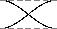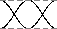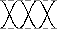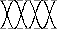2. Use the chart to find a formula for the wavelength in terms of the number of nodes.

The formula is different because there are more anti-nodes than nodes. The right formula is:

${\lambda }_{n}=\frac{2L}{n}$

Here, $n$ is still the number of nodes.

An open organ pipe is 0,853 m long. The speed of sound in air is 345 m.s ${}^{-1}$ . Can this pipe play middle C? (Middle C has a frequency of about 262 Hz)

The main frequency of a note is the fundamental frequency. The fundamental frequency of the open pipe has one node.

1. $f=\frac{v}{\lambda }$

We need to find the wavelength first.

$\begin{array}{ccc}\hfill \lambda & =& \frac{2L}{n}\hfill \\ & =& \frac{2\left(0,853\right)}{1}\hfill \\ & =& 1,706\phantom{\rule{4pt}{0ex}}\mathrm{m}\hfill \end{array}$
2. $\begin{array}{ccc}\hfill f& =& \frac{v}{\lambda }\hfill \\ & =& \frac{345}{1,706}\hfill \\ & =& 202\phantom{\rule{4pt}{0ex}}\mathrm{Hz}\hfill \end{array}$

This is lower than 262 Hz, so this pipe will not play middle C. We will need a shorter pipe for a higher pitch.

A flute can be modeled as a metal pipe open at both ends. (One end looks closed but the flute has an embouchure , or hole for the player to blow across. This hole is large enough for air to escape on that side as well.)If the fundamental note of a flute is middle C (262 Hz) , how long is the flute? The speed of sound in air is 345 m.s ${}^{-1}$ .

We can calculate the length of the flute from $\lambda =\frac{2L}{n}$ but:

1. $\begin{array}{ccc}\hfill f& =& \frac{v}{\lambda }\hfill \\ \hfill 262& =& \frac{345}{\lambda }\hfill \\ \hfill \lambda & =& \frac{345}{262}=1,32\phantom{\rule{4pt}{0ex}}\mathrm{m}\hfill \end{array}$
2. $\begin{array}{ccc}\hfill \lambda & =& \frac{2L}{n}\hfill \\ & =& \frac{2L}{1}\hfill \\ \hfill L& =& \frac{1,32}{2}=0,66\phantom{\rule{4pt}{0ex}}\mathrm{m}\hfill \end{array}$

Now let's look at a pipe that is open on one end and closed on the other. This pipe has a node at one end and an antinode at the other. An example of a musicalinstrument that has a node at one end and an antinode at the other is a clarinet. In the activity you will find out how the wavelengths are affected.

## Investigation : waves in a pipe open at one end

This chart shows some standing waves in a pipe open at one end. The pipe (shown as dashed lines) has length L.

1. Fill in the:
• number of nodes
• number of anti-nodes
• wavelength in terms of $L$
The first and last waves are done for you.
 Wave Nodes Antinodes Wavelength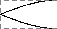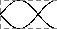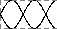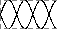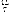2. Use the chart to find a formula for the wavelength in terms of the number of nodes.

The right formula for this pipe is:

${\lambda }_{n}=\frac{4L}{2n-1}$

A long wavelength has a low frequency and low pitch. If you took your pipe from the last example and covered one end, you should hear a much lower note! Also, the wavelengths of the harmonics for this tube are not integer multiples of each other.

A clarinet can be modeled as a wooden pipe closed on one end and open on the other. The player blows into a small slit on one end. A reed then vibrates in the mouthpiece. This makes the standing wave in the air. What is the fundamental frequency of a clarinet 60 cm long? The speed of sound in air is 345 m.s ${}^{-1}$ .

1. We are given:

$\begin{array}{ccc}\hfill L& =& 60\phantom{\rule{4pt}{0ex}}\mathrm{cm}\hfill \\ \hfill v& =& 345\phantom{\rule{4pt}{0ex}}\mathrm{m}.{\mathrm{s}}^{-1}\hfill \\ \hfill f& =& ?\hfill \end{array}$
2. $\begin{array}{ccc}\hfill \lambda & =& \frac{4L}{2n-1}\hfill \\ & =& \frac{4\left(0,60\right)}{2\left(1\right)-1}\hfill \\ & =& 2,4\phantom{\rule{4pt}{0ex}}\mathrm{m}\hfill \end{array}$
3. $\begin{array}{ccc}\hfill f& =& \frac{v}{\lambda }\hfill \\ & =& \frac{345}{2,4}\hfill \\ & =& 144\phantom{\rule{4pt}{0ex}}\mathrm{Hz}\hfill \end{array}$

This is closest to the D below middle C. This note is one of the lowest notes on a clarinet.

## Musical scale

The 12 tone scale popular in Western music took centuries to develop. This scale is also called the 12-note Equal Tempered scale. It has an octave divided into 12 steps. (An octave is the main interval of most scales. If you double a frequency, you have raised the note one octave.) All steps have equal ratios of frequencies. But this scale is not perfect. If the octaves are in tune, all the other intervals are slightly mistuned. No interval is badly out of tune. But none is perfect.

For example, suppose the base note of a scale is a frequency of 110 Hz ( a low A). The first harmonic is 220 Hz. This note isalso an A, but is one octave higher. The second harmonic is at 330 Hz (close to an E). The third is 440 Hz (also an A). Butnot all the notes have such simple ratios. Middle C has a frequency of about 262 Hz. This is not a simple multiple of 110 Hz. So the interval between C and A is a little out of tune.

Many other types of tuning exist. Just Tempered scales are tuned so that all intervals are simple ratios of frequencies.There are also equal tempered scales with more or less notes per octave. Some scales use as many as 31 or 53 notes.

What is a vector
vector is anything that has both a direction and a magnitude .they are usually drawn as pointed arrows ,the length of which represents a vector's magnitude
Tetteh
what is electronics?
Edward
how to calculate the reading on voltmeter or ammeter
why is HCl considered a strong acid
it dissociate almost completely
Tetteh
what is metal displacement
what is an electric field?
is the charge of an electron always 1,6 ×10^-19? and the mass is always 9,1×10^-13?
how to calculate a distance between charges
juss apply the formula of the Electrostatic force
Noluthando
please what's the simplest way to derived Schrodinger's wavelength equation
Maimo
how to show polarity
what can ii do to pass physics
Use previous years question papers to understand how questions are answered and asked
Joseph
but it hard ii am a slow learner
Slindile
Then the best thing to do is that immediately you are done reading through a certain topic, and you think you understood everything in that topic that's when you can use previous question papers and answer questions related to the topic. I think that's not difficult
Joseph
mm ii will try
Slindile
Good
Joseph
Here's a tip in reading textbooks, don't read it like a novel. First, flip through the pages—scan the chapter that you wanted to read. Second, go to the end of the chapter. Usually, there's a quiz at the end, so if will give you the important information that you need to know.
Sarah
Third, go to the beginning of the chapter and read through the words that were printed in bold: Titles, subtitles, headings, important words —because it helps to break down information.
Sarah
Fourth, read the first sentence of the chapter—if it is written by a good author; therefore, it will also have a good introduction. Check also the last sentence of the chapter to sum it up. Finally, read the whole chapter. You won't read it twice anymore.
Sarah
It looks hard, cause there are so much to do but read it thoroughly, it's easy and it will help you to save time and comprehend better. If you don't really have interest on reading—there are various of videos in youtube😊
Sarah
Oh, so em dashes turn into question marks :' (( nvm. Goodluck to all of us!
Sarah
How many ways can we calculate the empirical formula
How is current divided between resistors in parallel
Joseph
1/R=1/R+1/R
Slindile
tanx
Joseph
ii know 2 ways
Slindile
what's the other way
Joseph
What is the meaning of Coulomb's law
Electronic magnetic field
what is an emf ?
electromotive force
Tetteh
what are moments of a force
the moment of a force" is a measure of a tendency to cause a body to rotate about a specific point or axis....a moment is due to force not having equal and opposite force directly along its line of action.
Bhuboy
how to calculate the magnitude of the force of repulsionBy RhodesBy Stephen VoronBy OpenStaxBy Madison ChristianBy Sheila LopezBy OpenStaxByBy Caitlyn GobbleBy Jemekia WeedenBy Cath Yu